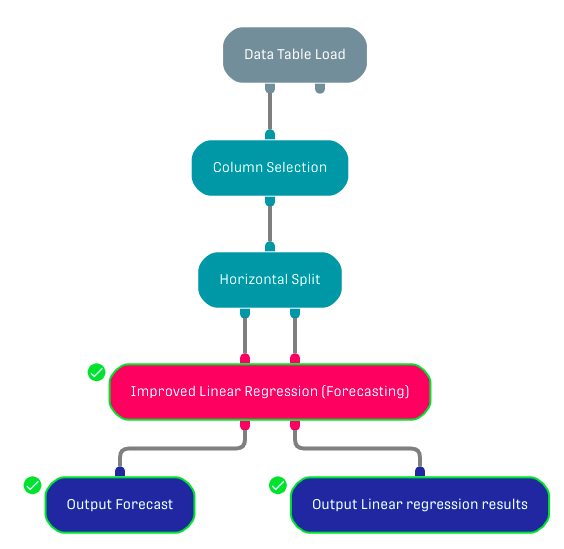# Overview

The processor performs a linear regression with one dependent and one or multiple independent variables.

The learning objective is to minimize the squared error, with regularization.

It is possible to use this processor without forecasting just by inserting a Replication Processor to enter the same input twice.

# Input

The processor requires two input datasets. The left input port corresponds to the training dataset (this data should be already labeled). The right input port corresponds to the test dataset.

The training and the test datasets must have the same schema.

# Output

The Improved Linear Regression Processor returns two different output tables:

• Output Forecast: Returns the input test dataset with an additional column containing the forecasted values.
• Output Linear Regression Results: Returns the regression statistics (weight, standard error, p-values, t-values).

In addition, the "Output Linear Regression Results" table is shown in the result tab within the processor along with other forecasting metrics.

# Example

In this example, the used data table contains YouTube videos relative information (total likes, dislikes, etc.). The processor of focus is used to forecast the number of comments.

## WorkflowThe Horizontal Split Processor is used to generate the training and test datasets from the original input. The Column Selection Processor is used to forward only the dependent and independent variables.

# Related Articles

Decision Tree Regression Forecast

Decision Tree Classification Forecast

Random Forest Classification Forecast Processor

Random Forest Regression Forecast Processor